Welcome to Lesson 9!Learning ObjectivesBy the end of this class, you should know...What is a tree and how does it differ from a linked list?What is a Binary Tree ADT?What are the properties of Binary Trees?How do you determine if a Binary Tree is balanced?1. Tree ADT1.1. Introducing TreesConsider the ADTs we have studied so far:ArrayLinked ListQueue StackThese structures all store data in a linear fashion, as in the example of a linked list show below:Image sourceHowever, storing data linearly is not the only option.In many cases, our data might not have a linear relationship.Consider, for example, a company's org. chart:Or, consider this depiction of the relationship among the countries in the world and their cities and states:Image source.Or, consider this data which is arranged into what we call a decision tree: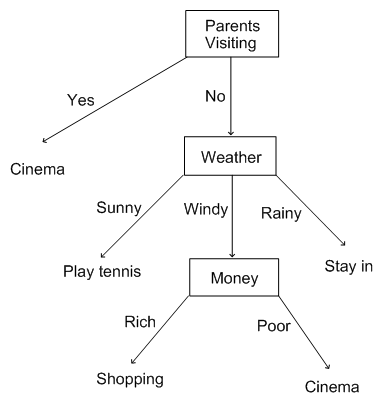Given the nature of certain types of data, sometimes it can make more sense to organize the data in hierarchical structure.Also, certain operations, such as search and insertion or deletion can be more efficient when we organize data hierarchically rather than linearly.In computer science, we can use a tree ADT to organize data hierarchically.1.2. The Structure of the Tree ADTLike linked lists, trees are comprised of nodes.These nodes store data and pointers to other nodes in the tree.Like the head and tail of a linked list, trees have specially-named nodes that serve important functions in the tree.The most important node is known as the root.Like the head of a linked list, a root is what allows us to access the first node in the tree.You can visualize a tree ADT with its root node like this:Image sourceTrees in computer science are usually depicted with the root node on top.Therefore, you have to visualize the tree upside down.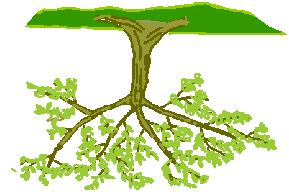Image sourceThe branches of the tree are called links or edges.Unless the tree is empty or has size==1, each Node in the tree is connected to other nodes by these pointers (links).Nodes in a tree have parent-child relationships.Each node can have only one parent node. But a node can have 0 or more child nodes.The root node in the image below has four child nodes.The root is considered the parent node of those four child nodes.For example, A is considered the child node of the root and the root is considered the parent node of A.Image sourceIn other words, the terminology we use to describe trees and their nodes, is similar to that of a family tree.Nodes in a tree can have parents, grandparents, cousins, siblings, children and grandchildren.They can also have ancestors and descendants.For example, in the tree below, Nodes 1,4,7, and 10 and their parents are all descendants of the the root node 5.The root node 5 is thus their ancestor.Image sourceAnother important node in a tree is the leaf node.The leaf node is any node in the tree with no children.In other words, the links of a leaf node point to Null.Any node that is not the root or a leaf is called an interior node as depicted above.The root and leaves of the tree are exterior nodes.1.3. Properties of TreesConsider a tree with n nodes. How many edges will it contain.Exactly n-1. Why is this the case?The reason is that all nodes, except the root, have exactly one incoming edge.The depth of a node X in a tree is defined as the number of edges from the root to the node X.What is the depth of the root node, given this definition?The height of a node X is defined as the number of edges in the longest path from the node X down to a leaf node.What is the height of any leaf node given this definition?Trees themselves can also have heights. The height of any tree is the same as the height of its root.Image source.1.4. Reflection: Labeling a Tree Given the tree below, answer the following questions:Image source.Step 1: Label the root and leaf nodesStep 2: List at least one of each of the following relationships:Parent-childSiblingsAncestorDescendantStep 3: identify 3 interior nodesStep 4: label the depth of the following nodes:htmlbodyliStep 5: label the height of the same nodes.2. The Binary Tree ADT2.1. Defining a Binary TreeA binary tree is a tree where each node can have at most 2 children.Below is an example of a binary tree. Note that each node has 0, 1 or 2 children.Image sourceHere is another example of a valid binary tree. Why does this tree meet the definition of a binary tree?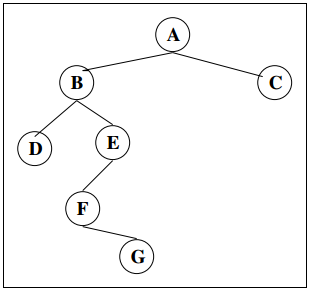Image source.Reflection: Is the below image a valid BT? Why or Why Not? Also, what does it resemble?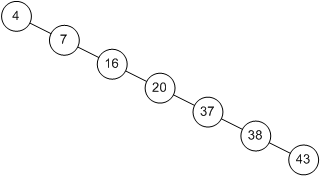These two children are called the left child and the right child.For example, in the BT above, 4 is the left child of its parent node 6 and 7 is the right child of its parent node 6.14 only has a left child, but does not have a right child.We can consider binary trees to be recursive structures.The root node links to root nodes of its left and right subtrees.These roots of the subtrees in turn link to the root nodes of their left and right subtrees.We will discuss this concept more when we get to Binary Search Trees.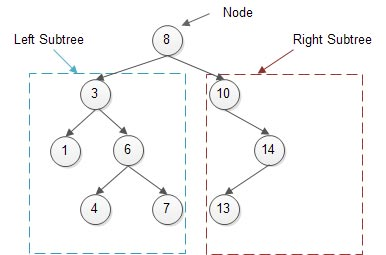Therefore, each node in the tree will contain some data as well as two references, one to the left child and one to the right child as depicted below:Image sourceIf a node has no right or left child, these values are set to Null.As you might have predicted, when we go to implement the binary search tree, we will be representing its node as a private inner class like this:private class Node{    T data;    Node left;    Node right;}Therefore, a Node has three fields:a field for the dataa field for the address of the left child (another Node).a field for the address of the right child (another Node).A binary tree is made up of Nodes, each with pointers to a left and right child node as shown below: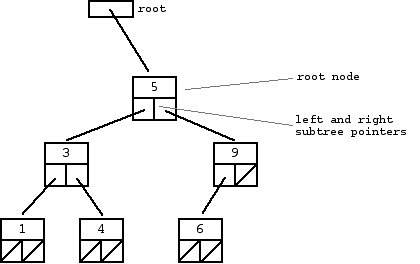Image source2.2 Special Types of Binary TreesA binary tree is considered a proper or strict binary tree if each node has either 0 or 2 children. Below is an example of a strict binary tree.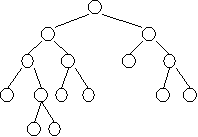Image source.A complete binary tree is one in which all levels are filled (except the last) and all nodes are as far left as possible. Below is an example of a complete binary tree.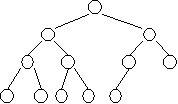Image source.A perfect or full binary tree is one in which all levels are completely filled.Image source.2.3. Balanced Binary TreesThe above trees are examples of balanced trees.In balanced trees, operations will be more efficient - as we shall discuss later.But what does balanced mean?There are several ways to define a balanced tree. We shall use the definition from a special type of balanced tree known as an AVL tree.A balanced binary tree is one in which the difference between the height of the left and right subtrees is not more than 1.| heightleft - heightright| <= 1 Let's give this a try.The below tree is balanced even though it might not appear so. Why?Consider the height of the right and left subtree of the root node A.hleft = 1 (height of B)hright = 2 (height of C)|1 - 2| = 1 <--balanced!Let's check another node, B.hleft = -1 (height of a null is defined to be -1) hright = 0 (height of D)|-1 - 0| = 1 <--balanced!2.4. ReflectionIs the binary tree depicted below balanced? Why or why not?Image source.Wrap UpAnswer the review questions on Canvas for today's lesson